# One-parameter subgroup

(diff) ← Older revision | Latest revision (diff) | Newer revision → (diff)

of a Lie groupover a normed fieldAn analytic homomorphism of the additive group of the fieldinto, that is, an analytic mapping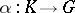such that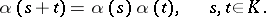The image of this homomorphism, which is a subgroup of, is also called a one-parameter subgroup. If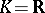, then the continuity of the homomorphismimplies that it is analytic. Ifor, then for any tangent vector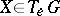toat the pointthere exists a unique one-parameter subgrouphavingas its tangent vector at the point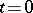. Here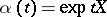,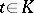, where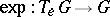is the exponential mapping. In particular, any one-parameter subgroup of the general linear group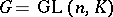has the form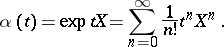Ifis a real Lie group endowed with a two-sidedly invariant pseudo-Riemannian metric or affine connection, then the one-parameter subgroups ofare the geodesics passing through the identity.

How to Cite This Entry:
One-parameter subgroup. Encyclopedia of Mathematics. URL: http://encyclopediaofmath.org/index.php?title=One-parameter_subgroup&oldid=11235
This article was adapted from an original article by r group','../u/u095350.htm','Unitary transformation','../u/u095590.htm','Vector bundle, analytic','../v/v096400.htm')" style="background-color:yellow;">A.L. Onishchik (originator), which appeared in Encyclopedia of Mathematics - ISBN 1402006098. See original article